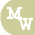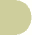calculate rate based on timenursing calculators search site map
 time = hours time = minutes time volume mL rate mL/hour

Examples

1)  If an order was written to infuse a liter of IV fluid every 8 hours, at what rate would the IV pump be set for? Answer is 125 mL/hour.

Using the calculator, select the time to equal hours (it's already preselected).  Next enter 8 as the value for time (8 hours).  Enter 1000 mL (1 L = 1000 mL) as the volume to be infused.  Click the "solve" button which calculates the result as 125 mL/hour.  See the example below.2)  You have recieved a new admission from the E.R.  The patient has class IV CHF and the doctor has ordered a loading dose of Inocor® (amrinone lactate).  The loading dose is to be administered over 3 minutes.  Pharmacy has brought the loading dose as a 50 mL IVPB.  At what rate should the IVPB be infused? Answer is 1000 mL/hour.

Using the calculator, select time to equal minutes.  Next, enter 3 as the value for time (3 minutes).  Enter 50 as the volume to be infused.  Click the "solve" button which calculates the result as 1000 mL/hour.  See the example below.Formulas

To calculate rate using hours

 VOLUME HOURS = mL/hr

To calculate rate using minutes

 VOLUME      (MINUTES / 60) = mL/hr